Next: Resistors in Series and Up: Electric Current Previous: Resistance and Resistivity

## Emf and Internal Resistance

Now, real batteries are constructed from materials which possess non-zero resistivities. It follows that real batteries are not just pure voltage sources. They also possess internal resistances. Incidentally, a pure voltage source is usually referred to as an emf (which stands for electromotive force). Of course, emf is measured in units of volts. A battery can be modeled as an emf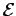connected in series with a resistor, which represents its internal resistance. Suppose that such a battery is used to drive a current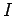through an external load resistor, as shown in Fig. 17. Note that in circuit diagrams an emfis represented as two closely spaced parallel lines of unequal length. The electric potential of the longer line is greater than that of the shorter one by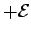volts. A resistor is represented as a zig-zag line.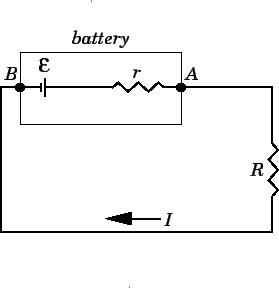Consider the battery in the figure. The voltageof the battery is defined as the difference in electric potential between its positive and negative terminals: i.e., the pointsand, respectively. As we move fromto, the electric potential increases byvolts as we cross the emf, but then decreases by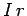volts as we cross the internal resistor. The voltage drop across the resistor follows from Ohm's law, which implies that the drop in voltage across a resistor, carrying a current, is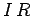in the direction in which the current flows. Thus, the voltageof the battery is related to its emfand internal resistancevia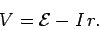(133)

Now, we usually think of the emf of a battery as being essentially constant (since it only depends on the chemical reaction going on inside the battery, which converts chemical energy into electrical energy), so we must conclude that the voltage of a battery actually decreases as the current drawn from it increases. In fact, the voltage only equals the emf when the current is negligibly small. The current draw from the battery cannot normally exceed the critical value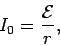(134)

since for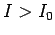the voltagebecomes negative (which can only happen if the load resistoris also negative: this is essentially impossible). It follows that if we short-circuit a battery, by connecting its positive and negative terminals together using a conducting wire of negligible resistance, the current drawn from the battery is limited by its internal resistance. In fact, in this case, the current is equal to the maximum possible current.

A real battery is usually characterized in terms of its emf(i.e., its voltage at zero current), and the maximum currentwhich it can supply. For instance, a standard dry cell (i.e., the sort of battery used to power calculators and torches) is usually rated at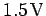and (say). Thus, nothing really catastrophic is going to happen if we short-circuit a dry cell. We will run the battery down in a comparatively short space of time, but no dangerously large current is going to flow. On the other hand, a car battery is usually rated at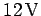and something like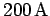(this is the sort of current needed to operate a starter motor). It is clear that a car battery must have a much lower internal resistance than a dry cell. It follows that if we were foolish enough to short-circuit a car battery the result would be fairly catastrophic (imagine all of the energy needed to turn over the engine of a car going into a thin wire connecting the battery terminals together).Next: Resistors in Series and Up: Electric Current Previous: Resistance and Resistivity
Richard Fitzpatrick 2007-07-14